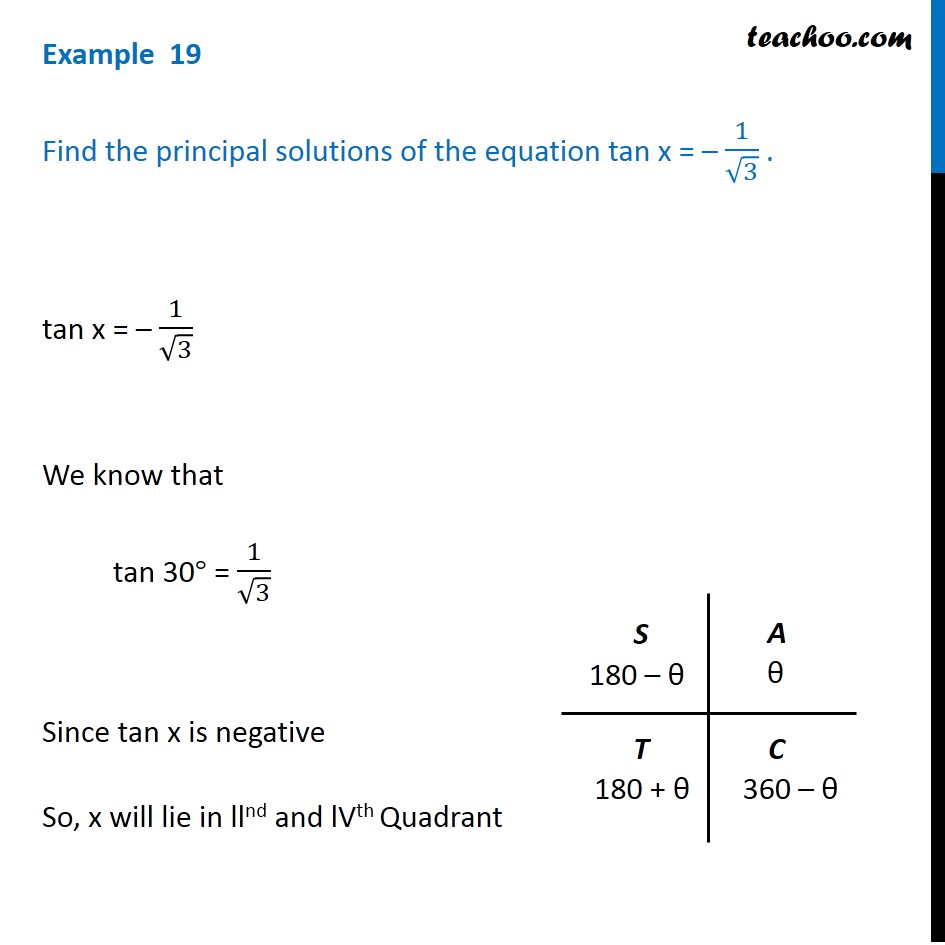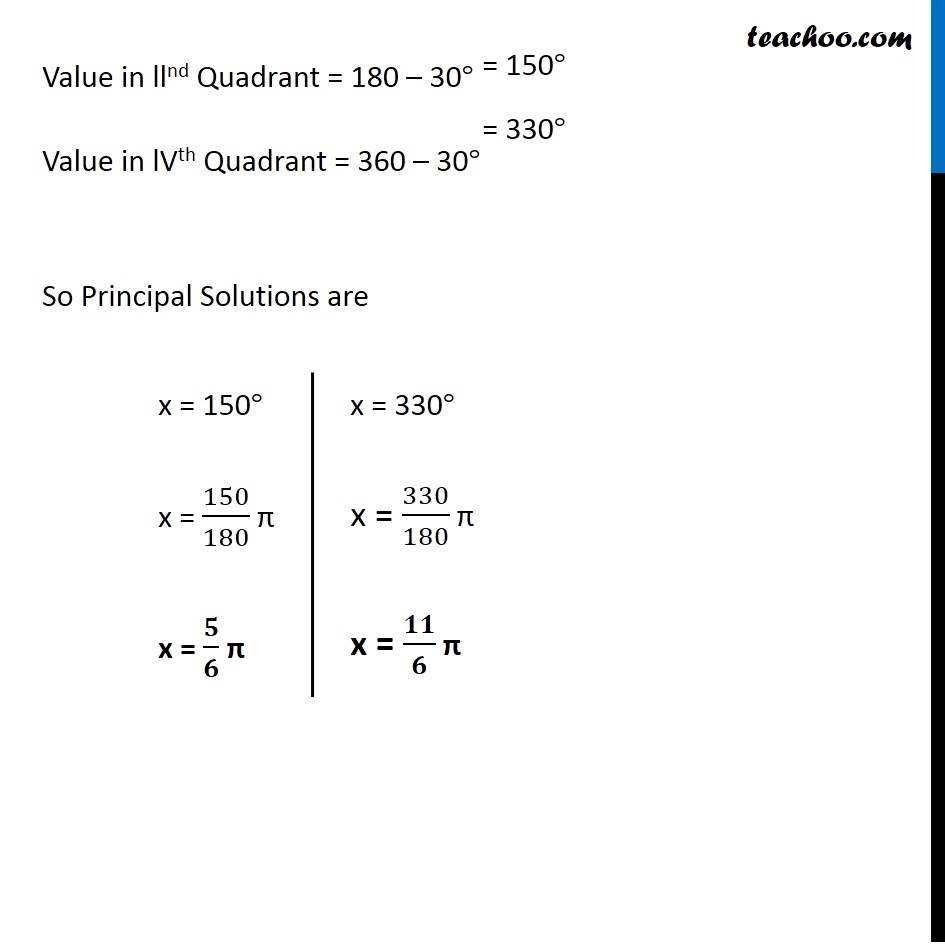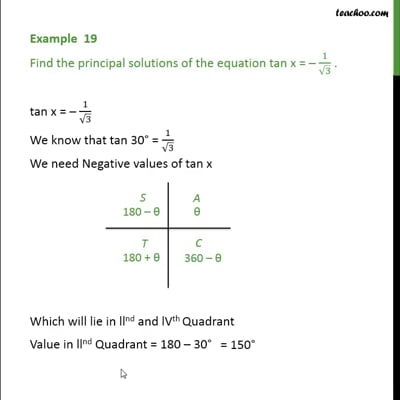Examples

Chapter 3 Class 11 Trigonometric Functions (Term 2)
Serial order wiseThis video is only available for Teachoo black users

### Transcript

Example 19 Find the principal solutions of the equation tan x = – 1/√3 . tan x = – 1/√3 We know that tan 30° = 1/√3 Since tan x is negative So, x will lie in llnd and lVth Quadrant Value in llnd Quadrant = 180 – 30° Value in lVth Quadrant = 360 – 30° So Principal Solutions are x = 150° x = 150/180 π x = 𝟓/𝟔 π x = 330° x = 330/180 π x = 𝟏𝟏/𝟔 π